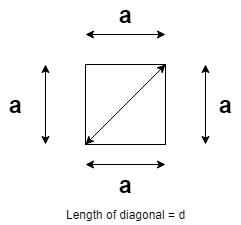Program to calculate length of diagonal of a square

• Difficulty Level : Medium
• Last Updated : 29 Apr, 2021

Given a positive integer S, the task is to find the length of diagonal of a square having sides of length S.

Examples:

Input: S = 10
Output: 14.1421
Explanation: The length of the diagonal of a square whose sides are of length 10 is 14.1421

Input: S = 24
Output: 33.9411

Approach: The given problem can be solved based on the mathematical relation between the length of sides of a square and the length of diagonal of a square as illustrated below:As visible from the above image, the diagonal and the two sides of the square form a right-angled triangle. Therefore, by applying Pythagoras Theorem:
(hypotenuse)2 = (base)2 + (perpendicular)2, where D and S are length of the diagonal and the square.

Therefore,
=>=>=>Therefore, simply calculate the length of the diagonal using the above-derived relation.

Below is the implementation of the above approach:

C++

 // C++ program for the above approach#include using namespace std; // Function to find the length of the// diagonal of a square of a given sidedouble findDiagonal(double s){    return sqrt(2) * s;} // Driver Codeint main(){    double S = 10;    cout << findDiagonal(S);     return 0;}

Java

 // Java program for the above approachimport java.util.*; class GFG{ // Function to find the length of the// diagonal of a square of a given sidestatic double findDiagonal(double s){    return (double)Math.sqrt(2) * s;} // Driver Codepublic static void main(String[] args){    double S = 10;         System.out.print(findDiagonal(S));}} // This code is contributed by splevel62

Python3

 # Python3 program for the above approachimport math # Function to find the length of the# diagonal of a square of a given sidedef findDiagonal(s):         return math.sqrt(2) * s # Driver Codeif __name__ == "__main__":     S = 10         print(findDiagonal(S)) # This code is contributed by chitranayal

C#

 // C# program for the above approachusing System;public class GFG{ // Function to find the length of the// diagonal of a square of a given sidestatic double findDiagonal(double s){    return (double)Math.Sqrt(2) * s;} // Driver Codepublic static void Main(String[] args){    double S = 10;         Console.Write(findDiagonal(S));}} // This code is contributed by 29AjayKumar

Javascript


Output:
14.1421

Time Complexity: O(1)
Auxiliary Space: O(1)

My Personal Notes arrow_drop_up